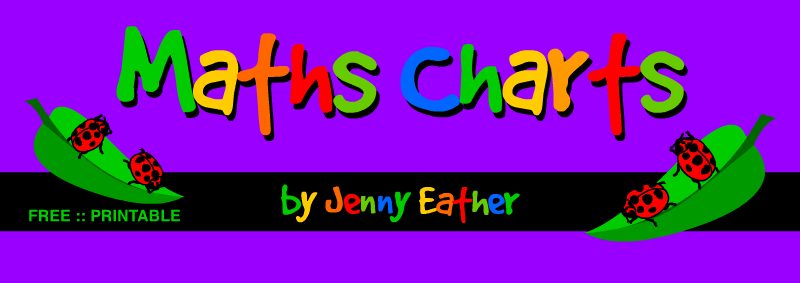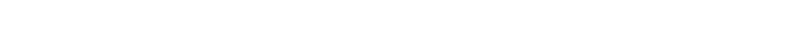maths charts ::||:: PRINTABLE ::||:: math posters Over 270 free printable maths charts or math posters suitable for interactive whiteboards, classroom displays, math walls, display boards, student handouts, homework help, concept introduction and consolidation and other math reference needs. ENTER HERE Mathematical areas covered include Numbers, Operations on Numbers, Fractions, Decimals, Percent and Percentages, Ratios and Rates, Beginning Algebra, Data and Statistics, Probability, Geometry, Measurement, Time and Money.© Jenny Eather. All rights reserved::| Math Charts | Math Terms | Math Words | Math Reference ::|:: Maths Charts | Maths Terms | Maths Words | Maths Reference |::
::| online math help | from a maths dictionary for kids | online maths help |::
::| printable math charts ::|| :: printable maths charts |::